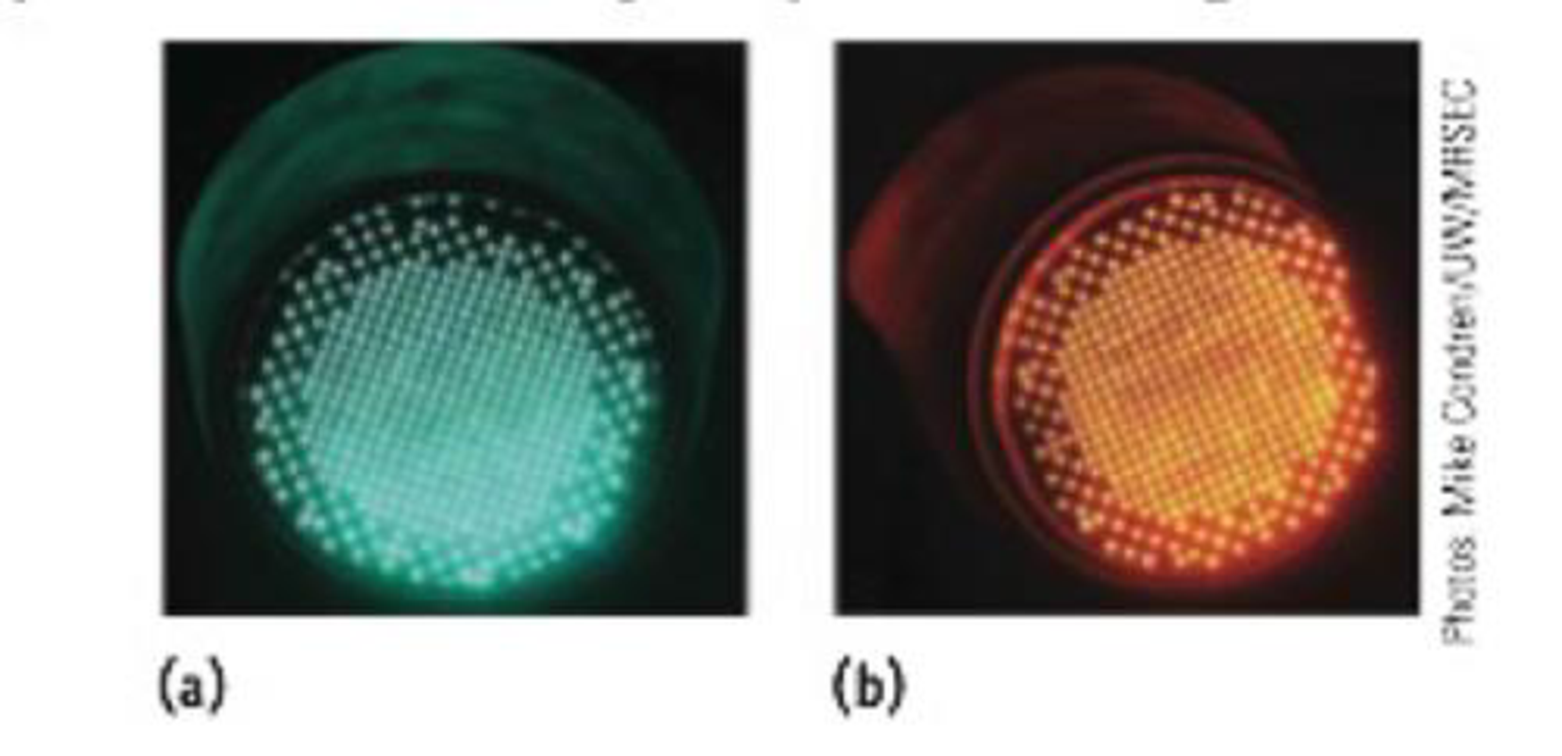Chapter 6, Problem 3PS

Chapter
Section
Textbook Problem

Traffic signals are often now made of LEDs (light-emitting diodes). Amber and green ones are pictured here. (a) The light from an amber signal has a wave-length of 595 nm, and that from a green signal has a wavelength of 500 nm. Which has the higher frequency? (b) Calculate the frequency of amber light.(a)

Interpretation Introduction

Interpretation: The light having higher frequency has to be determined among amber and green light.

Concept introduction:

• Electromagnetic radiations are a type of energy surrounding us. They are of different types like radio waves, IR, UV, X-ray etc.
• The visible light lies in the region between 400nm and 700nm.
• The frequency of the light is inversely proportional to its wavelength.

ν=cλwhere, c=speedoflightν=frequencyλ=wavelength

Explanation

The wavelength of amber light is 595nm and the wavelength of green light is 500nm. According to the relation between wavelength and frequency,ν </

(b)

Interpretation Introduction

Interpretation: The frequency of amber light has to be calculated.

Concept introduction:

The frequency of the light is inversely proportional to its wavelength.

ν=cλwhere, c=speedoflightν=frequencyλ=wavelength

Still sussing out bartleby?

Check out a sample textbook solution.

See a sample solution

The Solution to Your Study Problems

Bartleby provides explanations to thousands of textbook problems written by our experts, many with advanced degrees!

Get Started

Find more solutions based on key concepts